• +91 9971497814
• info@interviewmaterial.com

# RD Chapter 17- Constructions Ex-17.3 Interview Questions Answers

### Related Subjects

Question 1 : Construct a ∆ABC in which BC = 3.6 cm, AB + AC = 4.8 cm and ∠B = 60°.

Steps of construction :
(i) Draw a line segment BC = 3.6 cm.
(ii) At B, draw a ray BX making an angle of 60° and cut off BE = 4.8 cm.
(iii) Join EC.
(iv) Draw perpendicular bisector of CE which intersects BE at A.
(v) Join AC.
∆ABC is the required triangle.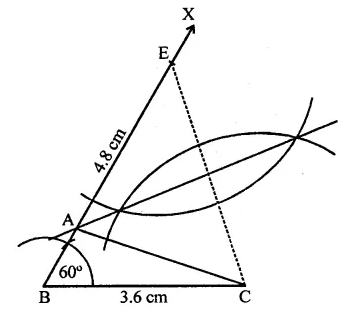Question 2 : Construct a ∆ABC in which AB + AC = 5.6 cm, BC = 4.5 cm and ∠B = 45°.

Steps of construction :
(i) Draw a line segment BC = 4.5 cm.
(ii) At B, draw a ray BX making an angle of 45° and cut off BE = 5.6 cm and join CE.
(iii) Draw the perpendicular bisector of CE which intersects BE at A.
(iv) Join AC.
∆ABC is the required triangle.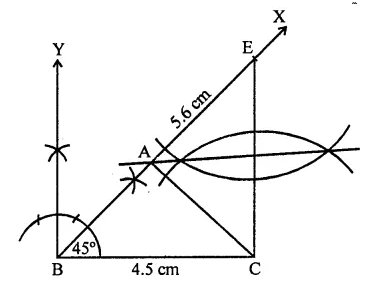Question 3 : Construct a AABC in which BC = 3.4 cm, AB – AC = 1.5 cm and ∠B = 45°.

Steps of construction :
(i) Draw a line segment BC = 3.4 cm.
(ii) At B, draw a ray BX making an angle of 45° and cut off BE = 1.5 cm.
(iii) Join EC.
(iv) Draw the perpendicular bisector of CE which intersects BE produced at A.
(v) Join AC.
∆ABC is the required triangle.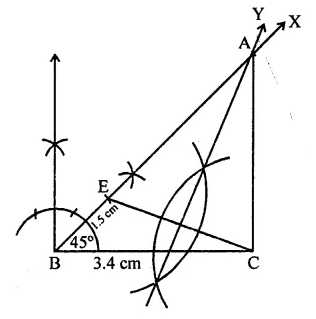Question 4 : Using ruler and compasses only, construct a ∆ABC, given base BC = 7 cm, ∠ABC = 60° and AB + AC = 12 cm.

Steps of construction :
(i) Draw a line segment BC = 7 cm!
(ii) At B, draw a ray BX making an angle of 60° and cut off BE = 12 cm.
(iii) Join EC.
(iv) Draw the perpendicular bisector of EC which intersects BE at A.
(v) Join AC.
∆ABC is the required triangle.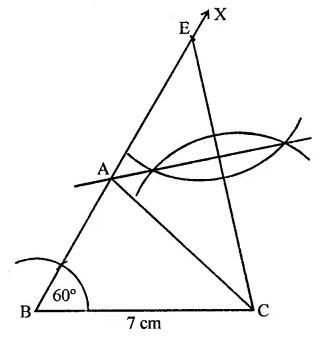Question 5 : Construct a right-angled triangle whose perimeter is equal to 10 cm and one acute angle equal to 60°.

Steps of construction :
(i) Draw a line segment PQ = 10 cm.
(ii) At P, draw a ray PX making an angle of 90° and at Q, QY making an angle of 60°.
(iii) Draw the angle bisectors of ∠P and ∠Q meeting each other at A.
(iv) Draw the perpendicular bisectors of AP and AQ intersecting PQ at B and C respectively,
(v) Join AB and AC.
∆ABC is the required triangle.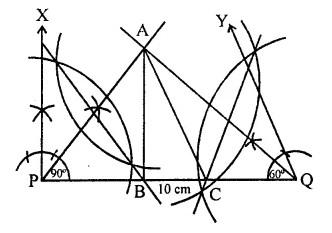Question 6 : Construct a triangle ABC such that BC = 6cm, AB = 6 cm and median AD = 4 cm.

Steps of construction :
(i) Draw a line segment BC = 6 cm and bisect it at D.
(ii) With centre B and radius 6 cm and with centre D and radius 4 cm, draw arcs intersecting each other at A.
(iii) Join AD and AB and AC.
Then ∆ABC is the required triangle.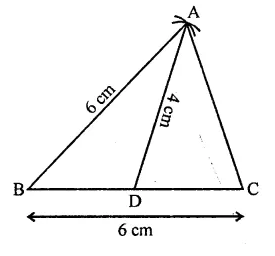Question 7 : Construct a right triangle ABC whose base BC is 6 cm and the sum of hypotenuse AC and other side AB is 10 cm.

Steps of construction :
(i) Draw a line segment BC = 6 cm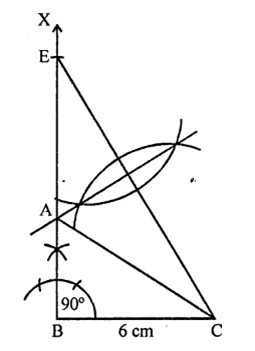(ii) At B, draw a ray BX making an angle of 90° and cut off BE = 10 cm.
(iii) Join EC and draw the perpendicular bisector of CE which intersects BE at A.
(iv) Join AC.
∆ABC is the required triangle.

Question 8 : Construct a triangle whose perimeter is 6.4 cm, and angles at the base are 60° and 45°.

Steps of construction :
(i) Draw a line segment PQ = 6.4 cm.
(ii) At P draw a ray PX making an angle of 60° and at Q, a ray QY making an angle of 45°.
(iii) Draw the bisector of ∠P and ∠Q meeting each other at A.
(iv) Draw the perpendicular bisectors of PA and QA intersecting PQ at B and C respectively.
(v) Join AB and AC.
∆ABC is the required triangle.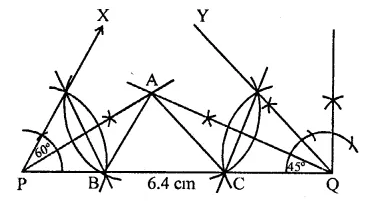Question 9 :
Using ruler and compasses only, construct a ∆ABC, from the following data:
AB + BC + CA = 12 cm, ∠B = 45° and ∠C = 60°.

Steps of construction :
(i) Draw a line segment PQ = 12 cm.
(ii) Draw ray PX at P making are angle of 45° and at Q, QY making an angle of 60°.
(Hi) Draw the angle bisectors of ∠P and ∠Q meeting each other at A.
(v) Draw the perpendicular bisector of AP and AQ intersecting PQ at B and C respectively.
(v) Join AB and AC.
∆ABC is the required triangle.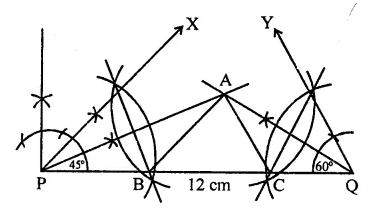Question 10 : Construct a triangle XYZ in which ∠Y = 30° , ∠Z = 90° XY +YZ + ZX = 11

Steps of construction :
(i) Draw a line segment PQ =11 cm.
(ii) At P, draw a ray PL making an angle of 30° and Q, draw another ray QM making an angle of 90°.
(iii) Draw the angle bisector of ∠P and ∠Q intersecting each other at X.
(iv) Draw the perpendicular bisector of XP and XQ. Which intersect PQ at Y and Z respectively.
(v) Join XY and XZ.
Then ∆XYZ is the required triangle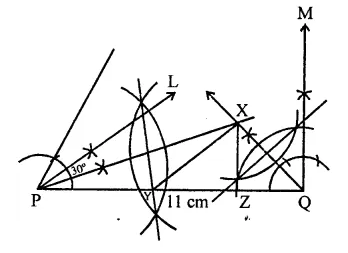Todays Deals### RD Chapter 17- Constructions Ex-17.3 Contributorskrishan

Name:
Email:

# Latest News# 9000 interview questions in different categories